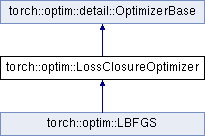Caffe2 - C++ API A deep learning, cross platform ML framework
torch::optim::LossClosureOptimizer Class Referenceabstract

Optimizer that requires the loss function to be supplied to the `step()` function, as it may evaluate the loss function multiple times per step. More...

`#include <optimizer.h>`

Inheritance diagram for torch::optim::LossClosureOptimizer:## Public Types

using LossClosure = std::function< Tensor()>
A loss function closure, which is expected to return the loss value.

## Public Member Functions

virtual Tensor step (LossClosure closure)=0Public Member Functions inherited from torch::optim::detail::OptimizerBase
OptimizerBase (std::vector< Tensor > parameters)
Constructs the `Optimizer` from a vector of parameters.

void add_parameters (const std::vector< Tensor > &parameters)
Adds the given vector of parameters to the optimizer's parameter list.

Zeros out the gradients of all parameters.

const std::vector< Tensor > & parameters () const noexcept
Provides a const reference to the parameters this optimizer holds.

std::vector< Tensor > & parameters () noexcept
Provides a reference to the parameters this optimizer holds.

size_t size () const noexcept
Returns the number of parameters referenced by the optimizer.

virtual void save (serialize::OutputArchive &archive) const
Serializes the optimizer state into the given `archive`.

Deserializes the optimizer state from the given `archive`.Protected Member Functions inherited from torch::optim::detail::OptimizerBase
template<typename T >
Tbuffer_at (std::vector< T > &buffers, size_t index)
Accesses a buffer at the given index. More...

Tensorbuffer_at (std::vector< Tensor > &buffers, size_t index)
Accesses a buffer at the given index, converts it to the type of the parameter at the corresponding index (a no-op if they match). More...Protected Attributes inherited from torch::optim::detail::OptimizerBase
std::vector< Tensorparameters_
The parameters this optimizer optimizes.

## Detailed Description

Optimizer that requires the loss function to be supplied to the `step()` function, as it may evaluate the loss function multiple times per step.

Examples of such algorithms are conjugate gradient and LBFGS. The `step()` function also returns the loss value.

Definition at line 110 of file optimizer.h.

The documentation for this class was generated from the following file: